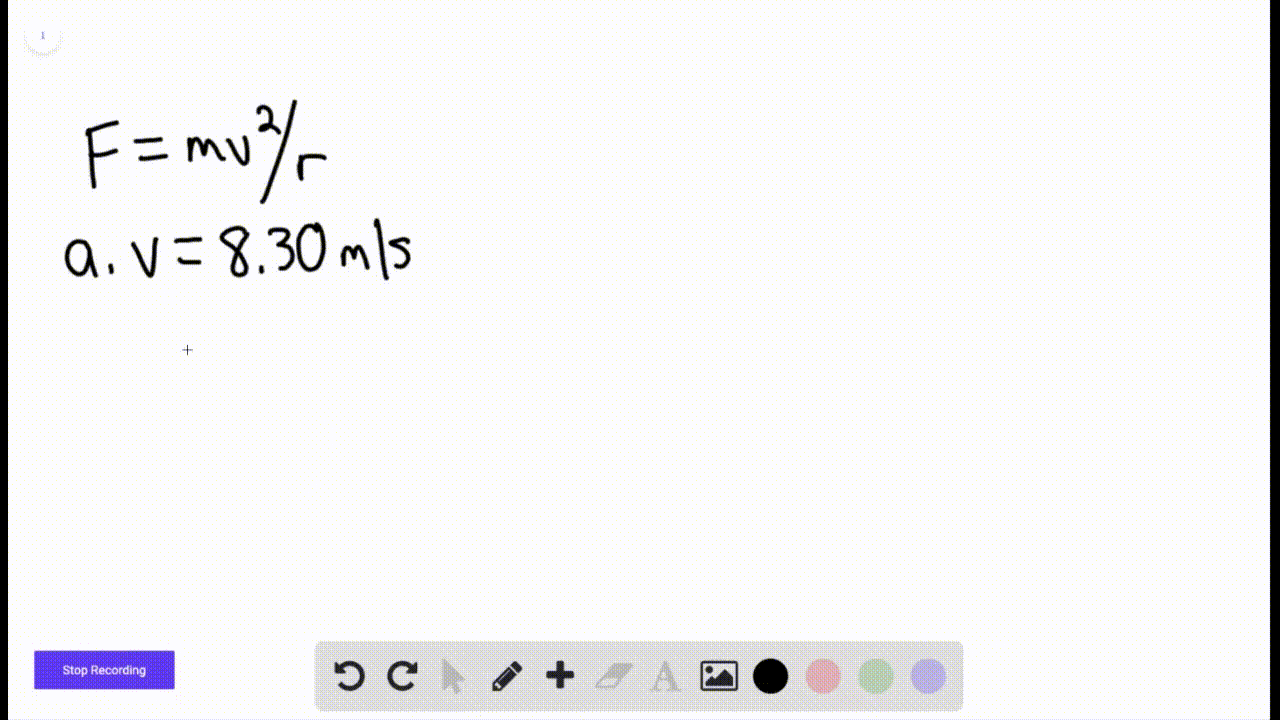Sign up for our free STEM online summer camps starting June 1st!View Summer Courses### An 85. 0 kg passenger is made to move along a cir…

03:40
AH
Carnegie Mellon University

Need more help? Fill out this quick form to get professional live tutoring.

Get live tutoring
Problem 49

In Fig. $6-39$ , a car is driven at constant speed over a circular hill and then into a circular valley with the same radius. At the top of the hill, the normal force on the driver from the car seat is 0 . The driver's mass is 70.0 $\mathrm{kg}$ . What is the magnitude of the normal force on the driver from the seat when the car passes through the bottom of the valley?

1372 $\mathrm{N}$

## Discussion

You must be signed in to discuss.

## Video Transcript

So here at the top of the hill, the situation is very similar to sample problem. The name of the same problem is called vertical circular loop a Diablo. And here, however, there's one change in that the normal force is reversed. And so we're simply going to then tweak our equation 6 19 and say that then the force normal is gonna be equaling. Um, multiplied by G minus v squared over r. And we know that here the normal forces equaling zero. Therefore, we can say that velocity squared equals G r and we can Then at the bottom of the Valley, we reverse both the normal force direction and the acceleration direction. So here at the bottom of the Valley again, we have the acceleration and the force normal reversed. So here we can say that the force normal, we'll be equaling m multiplied by G plus velocity squared over R. And so this is simply gonna be equaling 22 mg and this would be equaling two approximately 1370 Newtons. That is the end of the solution. Thank you for watching